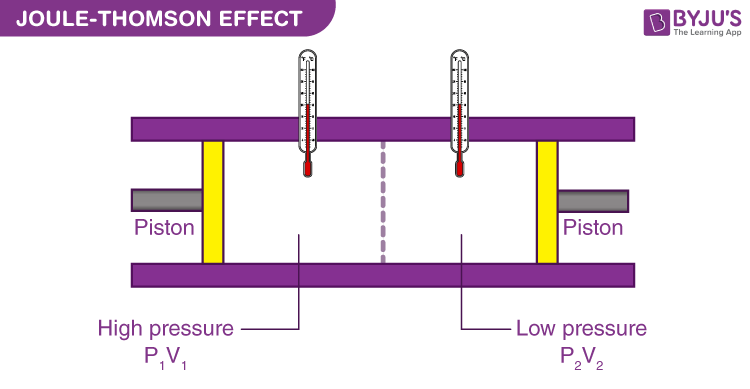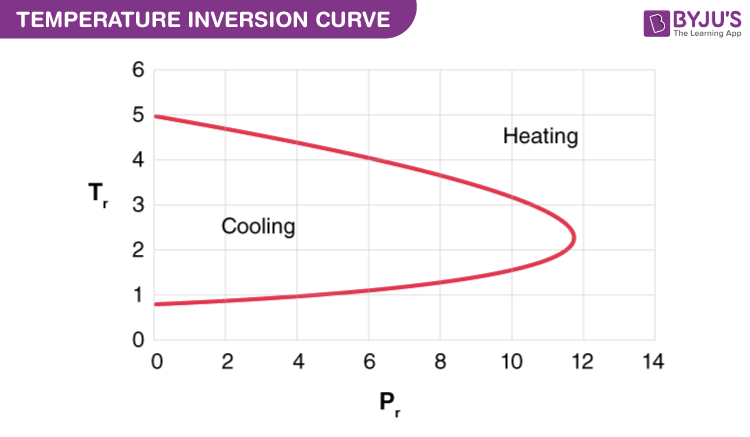# Joule-Thomson Effect

## What Is Joule-Thomson Effect?

The Joule-Thomson effect also known as Kelvin–Joule effect or Joule-Kelvin effect is the change in fluid’s temperature as it flows from a higher pressure region to lower pressure.According to the thermodynamic principle, the Joule-kelvin effect can be explained best by considering a separate gas packet placed in the opposite flow of direction for restriction. For the gas packet to pass through, the upstream gas needs to perform some work to push through the packet. The work equals the volume of the packet multiplied by the times of upstream pressure.

$$\begin{array}{l} W_{1}=V_{packet 1} \times P_{1} \end{array}$$

As the packet goes through the restriction, it has to make some room by displacing a considerable amount of the downstream gas. It includes performing the work which equals the product of packet volume and downstream pressure.

$$\begin{array}{l} W_{2}=V_{packet 2} \times P_{2} \end{array}$$

Due to the different effects of compressibility, the work performed upstream is not equal to the amount of work done downstream for real gases. Since depressuring is viewed as an adiabatic process, it reveals that any gas does not exchange work or heat by its surroundings, any change in internal energy has to follow the first law of thermodynamics.

$$\begin{array}{l} U_{2} – U_{1} = W_{1} – W_{2}\end{array}$$

Gas molecules are subjected to repulsive and attractive forces, (Van der Waals forces) as they are in random motion. When the gas pressure is lowered, i.e, the average distance between the molecules increases, the attractive forces become dominant for many gases at ambient temperature which results in an elevation in potential energy.

Most of the real gases need more work downstream at ambient temperature, due to the effects of compressibility.

$$\begin{array}{l} P_{1} \times V_{1} < P_{2} \times V_{2}\end{array}$$

This indicates that the internal energy decreases when the gas passes through the restriction.

It can be generalized that for many real gases, the temperature decreases during a reduction in pressure, but this is not true for every gas and condition. Depressuring is an isenthalpic process which reveals that enthalpy doesn’t change. The temperature can either decrease or increase for any gas based on how the internal energy changes to maintain the enthalpy constant.

## Joule Thomson Coefficient

$$\begin{array}{l}\mu _{JT}=(\frac{\partial T}{\partial P})_{H}\end{array}$$

The following table explains when the Joule-Thomson effect cools or warms a real gas:

 If the gas temperature is then μJT is since ∂P is thus ∂ must be So the gas above the inversion temperature negative always negative positive warms below the inversion temperature positive always negative negative cools

### Derivation of Joule Thomson Coefficient

Joule Thomson coefficient can be derived using the thermodynamic relationships and is defined as the isenthalpic change in temperature in the fluid due to pressure drop is given as:

$$\begin{array}{l}\mu =(\frac{\partial T}{\partial P})_{H}\end{array}$$
$$\begin{array}{l}(\frac{\partial T}{\partial P})_{T}(\frac{\partial P}{\partial T})_{H}(\frac{\partial T}{\partial H})_{P}=-1\end{array}$$
$$\begin{array}{l}(\frac{\partial H}{\partial P})_{T}=-(\frac{\partial H}{\partial T})_{P}(\frac{\partial T}{\partial P})_{H}\end{array}$$
$$\begin{array}{l}(\frac{\partial H}{\partial P})_{T}=-C_{P}\mu\end{array}$$
$$\begin{array}{l}(\frac{\partial H}{\partial P})_{T}=[\tilde{v}-T(\frac{\partial \tilde{v}}{\partial T})_{P}]\end{array}$$
(enthalpy change in terms of pressure, temperature and volume)

$$\begin{array}{l}\mu =\frac{RT^{2}}{P_{C_{P}}}(\frac{\partial Z}{\partial T})_{P}\end{array}$$
$$\begin{array}{l}\mu =(\frac{\partial T}{\partial P})_{H}=\frac{1}{C_{P}}[T(\frac{\partial \tilde{v}}{\partial T})_{P}-\tilde{v}]=-\frac{1}{C_{P}}(\frac{\partial H}{\partial P})_{T}=\frac{RT^{2}}{P_{C_{P}}}(\frac{\partial Z}{\partial T})_{P}\end{array}$$

### Temperature InversionThe temperature of a gas at which the reduction in pressure does not lead to any change in temperature is known as inversion temperature.  The gas gets heated up on expansion and cools down below this temperature.

## Applications of Joule-Thomson Effect

• The cooling produced in the Joule-Thomson expansion has made it a very valuable tool in refrigeration.
• The effect is applied in the Linde technique in the petrochemical industry, where the cooling effect is used to liquefy gases.
• It is also used in many cryogenic applications. For example for the production of liquid nitrogen, oxygen, and argon.
• The  effect can also be used to liquefy even helium

### What is the basic principle of Joule Thomson effect?

The basic principle of Joule Thomson effect is based on the transfer of heat. Also, at ordinary temperature and pressure, all real gases undergo expansion and this phenomenon is used in the process of liquefying gases. But this does not hold good for hydrogen and helium.

### What is inversion temperature in Joule Thomson effect?

The inversion temperature in Joule Thomson effect is defined as the temperature in which the sign of the coefficient changes.

### What is the Joule Thomson coefficient for an ideal gas?

The Joule Thomson coefficient for an ideal gas is equal to zero as the enthalpy of the gas is dependent on the temperature.

### What is the maximum inversion temperature?

The maximum inversion temperature for hydrogen is 200 K and the maximum inversion temperature for helium is 24 K.

### Why is Joule Thomson not applicable to hydrogen gas?

Joule Thomson is applicable to hydrogen gas but at lower temperatures. This is because when they are at higher temperatures, they are close to behaving like an ideal gas.

Stay tuned with BYJU’S to know more about the Joule Thomson Effect along with applications of inverse temperature in real-time.

Test your knowledge on Joule Thomson effect